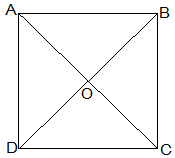Guru

# Show that the diagonals of a square are equal and bisect each other at right angles. Q.4

• 0

How i solve the question of class 9th ncert math of Quadrilaterals chapter of exercise 8.1 of question no 4 think it is very important question of class 9th give me the tricky way for solving this question  Show that the diagonals of a square are equal and bisect each other at right angles.

Share

1.Let ABCD be a square and its diagonals AC and BD intersect each other at O.

To show that,

AC = BD

AO = OC

and ∠AOB = 90°

Proof,

AB = BA (Common)

Thus,

AC = BD [CPCT]

diagonals are equal.

Now,

In ΔAOB and ΔCOD,

∠BAO = ∠DCO (Alternate interior angles)

∠AOB = ∠COD (Vertically opposite)

AB = CD (Given)

, ΔAOB ≅ ΔCOD [AAS congruency]

Thus,

AO = CO [CPCT].

, Diagonal bisect each other.

Now,

In ΔAOB and ΔCOB,

OB = OB (Given)

AO = CO (diagonals are bisected)

AB = CB (Sides of the square)

, ΔAOB ≅ ΔCOB [SSS congruency]

also, ∠AOB = ∠COB

∠AOB+∠COB = 180° (Linear pair)

Thus, ∠AOB = ∠COB = 90°

, Diagonals bisect each other at right angles

• 0
2.Let ABCD be a square and its diagonals AC and BD intersect each other at O.

To show that,

AC = BD

AO = OC

and ∠AOB = 90°

Proof,

AB = BA (Common)

Thus,

AC = BD [CPCT]

diagonals are equal.

Now,

In ΔAOB and ΔCOD,

∠BAO = ∠DCO (Alternate interior angles)

∠AOB = ∠COD (Vertically opposite)

AB = CD (Given)

, ΔAOB ≅ ΔCOD [AAS congruency]

Thus,

AO = CO [CPCT].

, Diagonal bisect each other.

Now,

In ΔAOB and ΔCOB,

OB = OB (Given)

AO = CO (diagonals are bisected)

AB = CB (Sides of the square)

, ΔAOB ≅ ΔCOB [SSS congruency]

also, ∠AOB = ∠COB

∠AOB+∠COB = 180° (Linear pair)

Thus, ∠AOB = ∠COB = 90°

, Diagonals bisect each other at right angles

• 0
3.Let ABCD be a square and its diagonals AC and BD intersect each other at O.

To show that,

AC = BD

AO = OC

and ∠AOB = 90°

Proof,

AB = BA (Common)

Thus,

AC = BD [CPCT]

diagonals are equal.

Now,

In ΔAOB and ΔCOD,

∠BAO = ∠DCO (Alternate interior angles)

∠AOB = ∠COD (Vertically opposite)

AB = CD (Given)

, ΔAOB ≅ ΔCOD [AAS congruency]

Thus,

AO = CO [CPCT].

, Diagonal bisect each other.

Now,

In ΔAOB and ΔCOB,

OB = OB (Given)

AO = CO (diagonals are bisected)

AB = CB (Sides of the square)

, ΔAOB ≅ ΔCOB [SSS congruency]

also, ∠AOB = ∠COB

∠AOB+∠COB = 180° (Linear pair)

Thus, ∠AOB = ∠COB = 90°

, Diagonals bisect each other at right angles

• 0